NEET  >  Graphs

# Graphs - Physics Class 11 - NEET

3. Graphs  :

(i) Straight line :
A linear relation between y & x represents a straight line.
General equation of straight line
y = mx + c
m ≡ slope of line
c ≡y intercept i.e. where the line cuts the y-axis.
Slope is defined as the tan of  angle made by the  straight line with positive x-axis in anticlockwise
direction.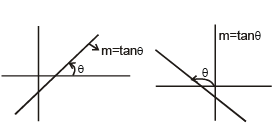m < 0 ⇒  θ > 90°
m > 0  ⇒ θ < 90°
0° ≤ θ < 180°

Ex.23 Draw the graph for the equation :   2y = 3x + 2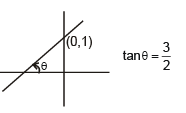Sol. 2y = 3x + 2  ⇒  y =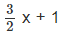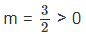⇒ θ < 90°
c = +1 > 0
⇒  The line will pass through (0, 1)

Ex.24 Draw the graph for the equation : 2y + 4x + 2 = 0
Sol.
2y + 4x + 2 = 0 ⇒ y = – 2x – 1
m = – 2 < 0  i.e., θ > 90°
c = – 1 i.e.,
line will pass through (0, –1)

•  (i) If c = 0 line will pass through origin.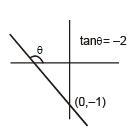(ii) y = c will be a line parallel to x axis.
(iii) x = c will be a line perpendicular to y axis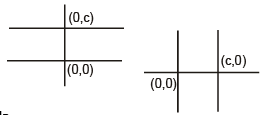(ii) Parabola
A general quadratic equation represents a parabola.
y = ax2 + bx + c a ≠ 0
if a > 0 ; It will be a opening  upwards parabola.
if a < 0 ; It will be a opening downwards parabola.
if c = 0 ; It will pass through origin.

e.g. y = 4 x2  + 3x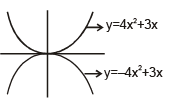Average velocity & instantaneous velocity from Position vs time graph
Average velocity from tto t
=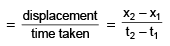=   tan θ  = slope of the chord AB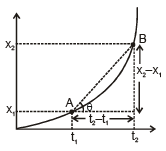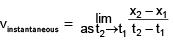when t2 approaches t1 point B approaches Point A and the chord AB becomes tangent to the curve. Therefore
vinstantaneous = Slope of the tangent x – t curve

(A) Reading x v/s t graphs

Explanation

(1)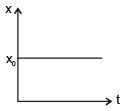Body is at rest at x0.

(2)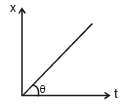Body starts from origin and is moving with speed tan θ away from origin.

(3)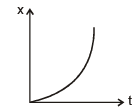Body starts from rest from origin and moves away from origin with increasing speed velocity and positive acceleration.

(4)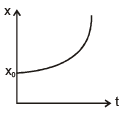Body starts from rest from x = x0 and moves away from origin with increasing  velocity or +ve acceleration.

(5)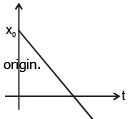Body starts from x = x0 and  is moving toward the origin with constant velocity passes throw origin after same time and continues to move away from origin.

(6)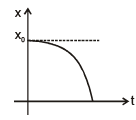Body starts from rest at x = x0 and then moves with increasing speed towards origin

∴ acceleration is –ve

7)  Body starts moving away from origin with some initial speed. Speed of  body is  decreasing till t1 and it becomes 0 momentarily of t = t1 and at this instant. Its reverses its direction  and move towards the origin with increasing speed.

(8)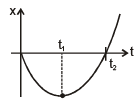Body starts from origin moves away from origin in the –ve x-axis at t = t1 with decreasing speed and at t= t1 it comes at rest momentarily, Reverses its direction  moves towards the origin the increasing speed. Crosses the origin at t = t2.

(9)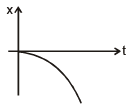Body starts from origin from rest and moves away from origin with increasing  speed.

(B) V-t Graphs

(1)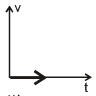Body is always at rest.

(2)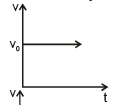Body is moving with constant velocity v0

(3)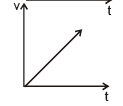Body is at rest initially then it starts moving with its velocity increasing at a  constant rate i.e. body is moving with constant acceleration.

(4)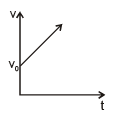Body starts its motion with initial velocity v0 and continues to move with its velocity  increasing at a constant rate i.e.  acceleration of the body is constant.

(5)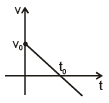Body starts its motion with initial velocity v0. Then it continues to move with its  velocity decreasing at a constant rate i.e. acceleration of the body is negative  and constant.  At t = t0 the body comes to rest instantaneously and reverses its direction of motion and then continues to move with decreasing velocity or increasing speed.
For 0 < t < t0    motion of the body is deaccelerated (∴ speed is decreasing)
t > t0  motion of the body is accelerated (∴ speed is increasing)

(6)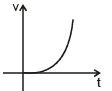Body is at rest initially. Then it starts moving with increasing velocity. As time  increases its velocity is increasing more rapidly. i.e. the moving with increasing  acceleration.

(7)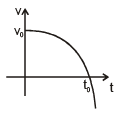Body starts its motion with initial velocity v0. Its  velocity is decreasing with time and at t = t0 . It becomes zero after body reverse its direction of motion and continues to move with decreasing velocity or increasing speed. Since velocity  of the body is decreasing for whole motion. Therefore, its acceleration is negative.For 0 < t < t0    motion of the  body is deaccelerated (speed is decreasing) t > t0  motion of the body is accelerated (Q speed is increasing)
(C) Reading of a - t Graphs

(1)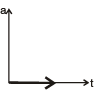acceleration of the body is zero that means the body is moving constant velocity.

(2)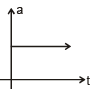Acceleration of the body is constant and positive.

(3)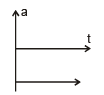Acceleration of the body is constant and negative

(4)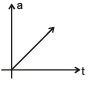Initially the acceleration of  the body is zero. Then its acceleration is increasing
at a constant rate.

(5)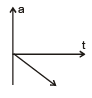The body starts accelerating(initial acceleration zero) at t = 0. Its acceleration is negative for whole of its motion and is decreasing at a constant rate.

(6)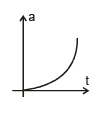Initially acceleration of the body is zero. Its acceleration is positive for whole of  its motion. Its acceleration is increasing for whole of its motion.

(IV) Drawing of graphs on the basis of given information.

(a) If acceleration of the body is zero.
(i) If the velocity of the body is v0 and it starts from origin.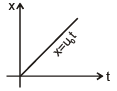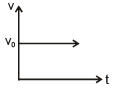(ii) If at t = 0, x = x0 then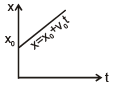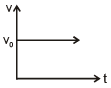(iii) If at t = 0,  x = – x0  then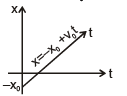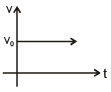b) If a body has constant acceleration :
For this section

(i) u0, x0 & a0 are positive constants.

(ii) uº initial velocity

(iii) vº  velocity at any time t.
(iv) xº Position at any time t.
xi º initial position

(i) if u = 0, a = a0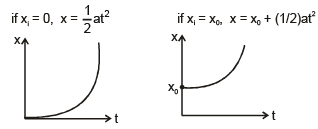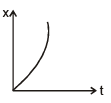This is wrong because it suggest the body don't have some initial velocity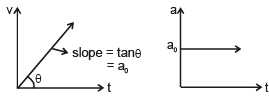v = a0t
(ii) If u = u0 ,  a = a0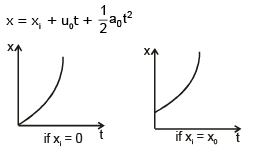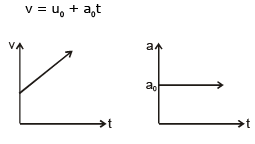(iii)   if u = u0,  a = – a0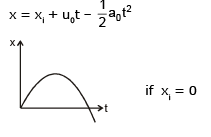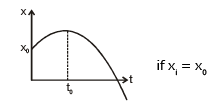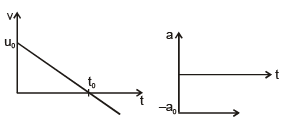iv) if u = – u0 ,  a = + a0
x = xi – u0t +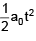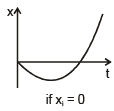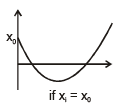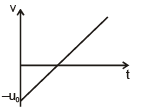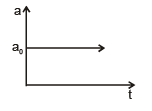(v) If u = u0,  a = – a0
x = xi – u0t –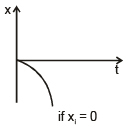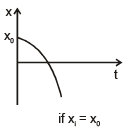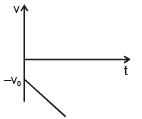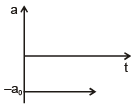Ex.25 Draw the
(a) position vs time graph
(b) velocity vs time graph
(c) acceleration vs time graph
for the following cases

(i) If a body is projected vertically upwards with initial velocity u. Take the projection point to be origin and upward direction as positive.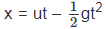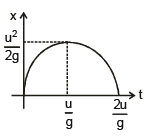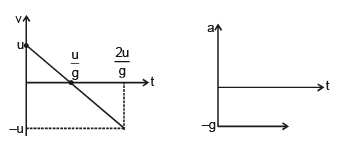(ii) If a body is dropped from a height h above the ground. Take dropping point to be origin and upward direction as +ve.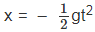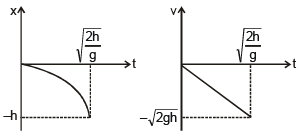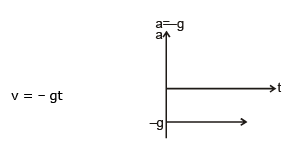(iii) If a body is projected vertically upwards from a tower of height h with initial velocity u. Take the projection point to be origin and upward direction as +ve.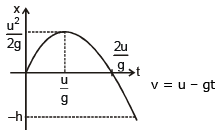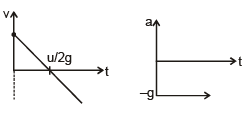(iv) A car starting from rest accelerates uniformly at 2 ms–2 for 5 seconds and then moves with constant speed acquired for the next 5 seconds and then comes to rest retarding at 2 ms–2.
Draw its
(a) Position vs time graph (b) Velocity vs time graph (c) acceleration vs time graph

acceleration vs time graph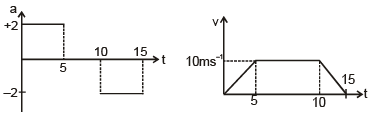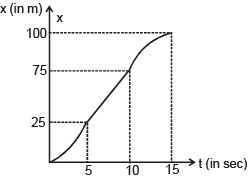acceleration vs time graph   velocity vs time graph Position vs time graph

(v) A particle starts from x = 0 and initial speed 10 ms–1 and moves with constant speed 10ms–1 for 20 sec. and then retarding uniformly comes to rest in next 10 seconds.
acceleration vs time graph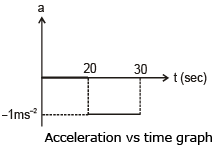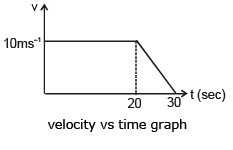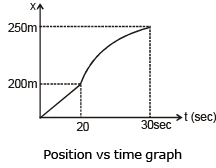(V) Conversion of velocity v/s time graph to speed v/s time graph.

As we know that magnitude of velocity represent speed therefore whenever velocity goes

–ve take its mirror image about time axis.
(VI) Conversion of displacement vs time graph to distance vs time graph   For distance time graph just make the mirror image of the displacement time graph from point of zero velocity onwards.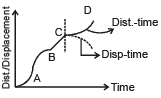(VII) Conversion of v - t graphs in to x-t and a-t graphs
(i)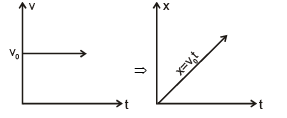(ii)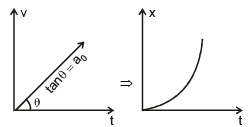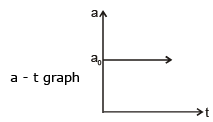(iii)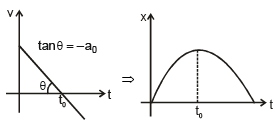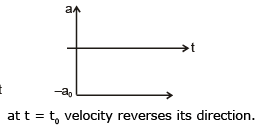(iv) x – t graph
From t = 0 to t = t1 acceleration = 0 therefore
from t = 0 to t = t1, x - t graph will be a straight line.
From t = t1 to t2 acceleration is negative

∴ It will be an opening downward parabola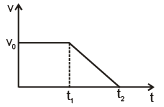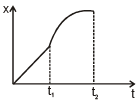(v) upto t = t1 acceleration is +ve
t1 < t < tacceleration is zero.
t > t2 acceleration is –ve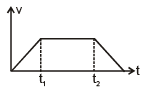x - t graph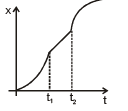Some important points :

•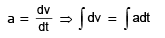⇒ Δv = area under the a - t curve

•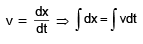⇒ Δx = area under the v - t curve

⇒ displacement = area under the v - t curve

Ex-26 If at t = 0 u = 5 ms–1 then velocity at t = 10 sec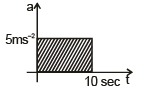= u + change in velocity
= 5 + area of the shaded part
= 5 + 10 × 5
= 55 ms–1

Ex-27 if at t = 0, u = 2 ms–2 find out it maximum velocity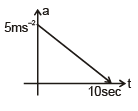Since whole motion is accelerating. Therefore velocity  will be max at the end of the motion which will be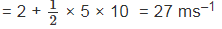Ex-28 if at t = 0, u = 4 ms–1
Find out v at
t = 10 sec,  t = 20 sec & t = 30 sec.
Since for whole motion acceleration of the body is positive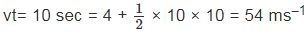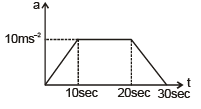∵ x2 > x1
∴ At the end of the motion A is at a greater distance from the starting point then B

Ex-29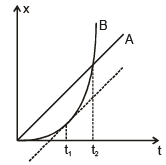Conclusions :

(i) Both A & B starts their motion at same time t = 0 and from same point x = 0.
(ii) Both are moving away from the starting point.
(iii) A is moving with constant velocity while B starts its motion from rest and its velocity is increasing with time i.e. it has some positive acceleration.
(iv) Q At t = t1 the tangent on B's graph becomes parallel to the A's graphs
∴ At t = t1 velocity of both A & B is same.
(v) For t < t1 velocity of A is greater than velocity of B. Therefore up to t = t1, separation between A & B increases with time.
(vi) For t > t1 velocity of B is greater than velocity of A. Therefore after t = t1 separation between A & B starts decreasing and it becomes zero at t = t2 where B overtakes A.

The document Graphs | Physics Class 11 - NEET is a part of the NEET Course Physics Class 11.
All you need of NEET at this link: NEET

## FAQs on Graphs - Physics Class 11 - NEET

 1. What are graphs in mathematics?Ans. In mathematics, graphs are visual representations of data that consist of nodes (also known as vertices) and edges (also known as arcs or lines) connecting these nodes. They are used to depict relationships between different elements or entities.
 2. How are graphs used in real-world applications?Ans. Graphs have numerous applications in various fields such as computer science, social networks, transportation, and biology. They can be used to model and analyze complex systems, optimize routes and networks, understand social connections, and study biological networks like protein interactions.
 3. What are the different types of graphs?Ans. There are several types of graphs, each serving a specific purpose. Some common types include: - Bar Graphs: Used to compare different categories or groups. - Line Graphs: Used to show trends or changes over time. - Pie Charts: Used to represent proportions or percentages. - Scatter Plots: Used to show the relationship between two variables. - Network Graphs: Used to visualize the connections between nodes.
 4. How do you interpret a graph?Ans. To interpret a graph, one should analyze its key components such as axes, labels, and units. It is essential to understand the variables being represented and the scale used in the graph. Additionally, examining the shape, trends, and patterns within the graph can provide insights into the data and help draw conclusions or make predictions.
 5. How can graphs help in data analysis?Ans. Graphs are valuable tools for data analysis as they provide a visual representation of data, making it easier to identify patterns, trends, and outliers. They can help in understanding the distribution of data, comparing different datasets, and presenting complex information in a concise and understandable manner. Additionally, graphs facilitate the communication of findings and insights to others.

## Physics Class 11

130 videos|483 docs|210 tests

## Physics Class 11

130 videos|483 docs|210 tests

### How to Prepare for NEET

Read our guide to prepare for NEET which is created by Toppers & the best Teachers
Signup to see your scores go up within 7 days! Learn & Practice with 1000+ FREE Notes, Videos & Tests.
10M+ students study on EduRev
Track your progress, build streaks, highlight & save important lessons and more!(Scan QR code)
Related Searches

,

,

,

,

,

,

,

,

,

,

,

,

,

,

,

,

,

,

,

,

,

;